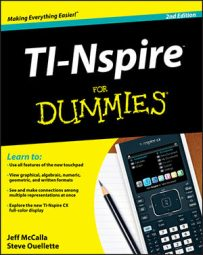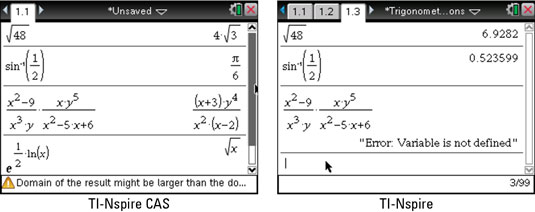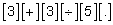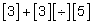##### TI-Nspire For DummiesTI-Nspire CAS returns results in symbolic form. That is, results are given as exact values — the way you typically see them in textbooks or other printed materials. Check out the first screen where some expressions have been entered by using a combination of primary and secondary keys. On the third line of this same screen, you can see that TI-Nspire CAS attempts to display algebraic expressions in simplified form.

In the case of the product of two rational expressions, common factors are divided out, and the result is displayed as a single, simplified rational expression. TI-Nspire CAS can even handle complicated expressions such as the one shown in the last line of the first screen.

Notice that warning message located at the bottom of the first screen. The complete message reads “Domain of the result might be larger than the domain of the input.” This message occurs because the domain of the input is the set of all real numbers greater than zero, whereas the domain of the output is the set of all real numbers greater than or equal to zero.As a comparison, the first three expressions have been typed on the TI-Nspire Handheld, as shown in the second screen. The first two results are given as decimal approximations. The rational expression on the third line returns an error message because the variables x and y are not defined and, therefore, do not have a numerical value.

If the numerical values were stored to x and y, TI-Nspire would return the numerical result of this expression evaluated at these stored values.

TI-Nspire and TI-Nspire CAS do not always display results as decimals. Answers that are rational numbers are almost always given in fractional form. However, the examples shown in the second screen have irrational answers and, therefore, must be given as decimal approximations.

At times, it is advantageous to view the decimal approximation of an answer. As you do with TI-Nspire, press [CTRL][ENTER] to evaluate an expression and force a result as a decimal. Here are three additional ways to obtain an approximate result:

• Include a decimal point somewhere in your calculation. For example, pressto evaluate the first example in decimal form.

• Use the Convert to Decimal command. Pressand then press [MENU]→Number→Convert to Decimal. This command can also be found in the Catalog.

• Use the Approx command to force a result in decimal form or scientific notation. This command can be typed using the alpha keys and can also be found in the Catalog.